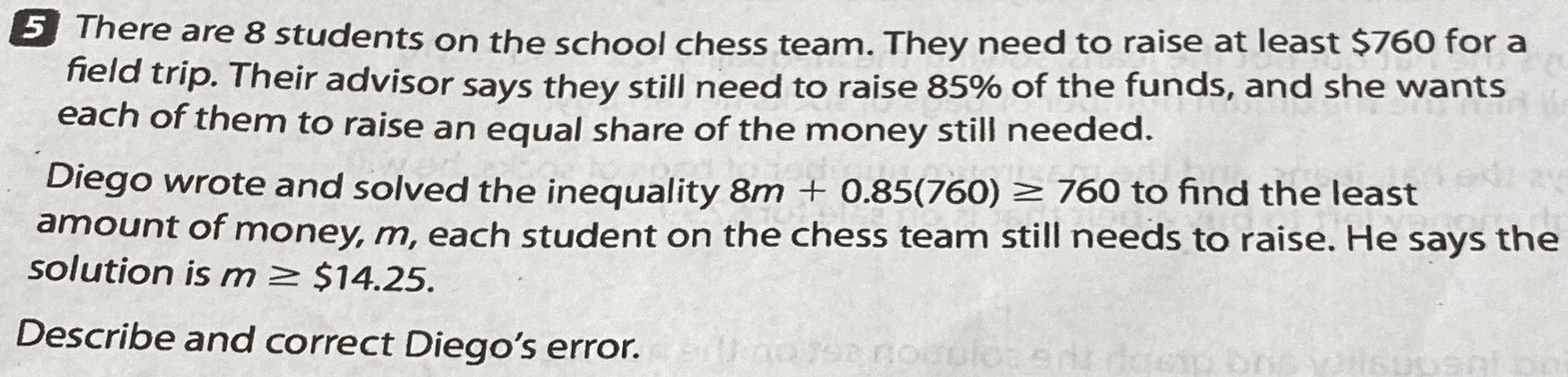### ¿Todavía tienes preguntas de matemáticas?

Pregunte a nuestros tutores expertos
Algebra
Pregunta$$5$$ There are $$8$$ students on the school chess team. They need to raise at least $$\ 760$$ for a field trip. Their advisor says they still need to raise $$85 \%$$ of the funds, and she wants each of them to raise an equal share of the money still needed. Diego wrote and solved the inequality $$8 m + 0.85 ( 760 ) \geq 760$$ to find the least amount of money, $$m$$ , each student on the chess team still needs to raise. He says the solution is $$m \geq \ 14.25$$ . Describe and correct Diego's error.

$$8 m \geq \frac { 85 } { 100 } \times 760$$
$$8 m \geq 646$$
$$m \geq \frac { 646 } { 8 }$$
$$m \geq 80.75$$